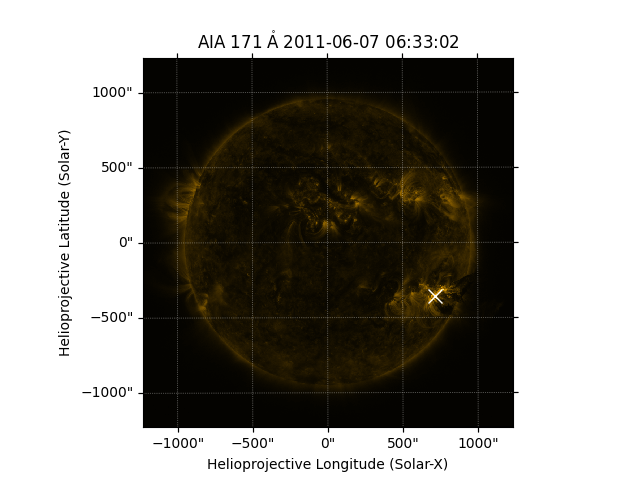# The brightest pixel location¶

How to find and overplot the location of the brightest pixel

from __future__ import print_function, division

import numpy as np
import astropy.units as u
import matplotlib.pyplot as plt

import sunpy.map
from sunpy.data.sample import AIA_171_IMAGE


We first create the Map using the sample data and import the coordinate functionality.

aia = sunpy.map.Map(AIA_171_IMAGE)

pixel_pos = np.argwhere(aia.data == aia.data.max())*u.pixel
hpc_max = aia.pixel_to_world(pixel_pos[:, 1], pixel_pos[:, 0])


Let’s now plot the results. We’ll overlay the autogenerated SunPy lon/lat grid as well for comparison.

fig = plt.figure()
ax = plt.subplot(projection=aia)
aia.plot()
ax.plot_coord(hpc_max, 'bx')
plt.show()Total running time of the script: ( 0 minutes 0.824 seconds)

Gallery generated by Sphinx-Gallery